# Weighted Average Profit Method | Class 12

Weighted Average Profit Method is the method of computing goodwill, where value of Goodwill is equal to the (Weighted Average Profit X Number of year’s purchase). Weighted Average Profit are calculated as under : –

• Assign  given weights to the profits of the respective years. Generally, higher weights are given to recent year profits.
• Multiply profits of respective year with the weights;
• Add all the Products so obtained
• Divide the sum of Products by number of years to get the Weighted Average Profit

Goodwill = Weighted Average Profit X Number of year’s purchase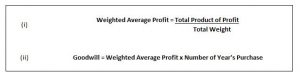Weighted Average Profit Method – Question 1 : –

The profits of a firm for the year ended 31st March for 2018 were as under :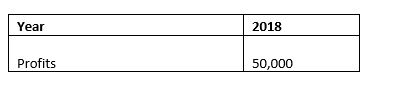What would be the value of goodwill on the basis of  1 years purchase of the weighted average profit of 2018 ?

Explanation : –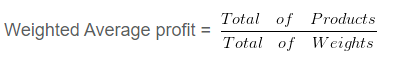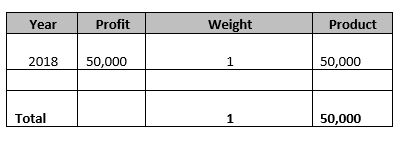Weighted Average profit = 50000/1
= 50,000

Goodwill = Weighted Average Profit X Number of year’s purchase

= 50,000 X 1
= Rs. 50,000

Weighted Average Profit Method – Question 2 : –

The profits of a firm for the year ended 31st March for the last 2 years were as under : –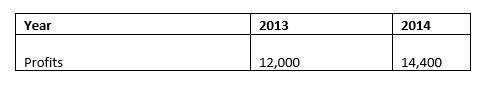What would be the value of goodwill on the basis of 3 years purchase of the weighted average profits of the last two years, assuming weight of 1 and 2 are assigned to 2013 and 2014 respectively ?

Explanation : –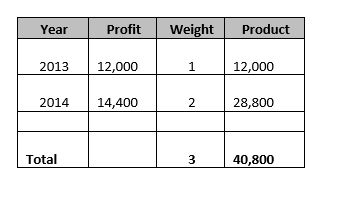Weighted Average profit = 40800/3
= 13,600

Goodwill = Weighted Average Profit X Number of year’s purchase
= 13,600 X 3
= Rs. 40,800

Learn More..